﻿ BP神经网络和支持向量机相结合的电容器介损角辨识
«上一篇文章快速检索 高级检索

 智能系统学报2019, Vol. 14Issue (1): 134-140  DOI: 10.11992/tis.2018050340

### 引用本文ZHAO Wenqing, YAN Hai, WANG Xiaohui. Capacitor dielectric loss angle identification based on a BP neural network and SVM[J]. CAAI Transactions on Intelligent Systems, 2019, 14(1): 134-140. DOI: 10.11992/tis.201805034.### 文章历史

BP神经网络和支持向量机相结合的电容器介损角辨识

Capacitor dielectric loss angle identification based on a BP neural network and SVM
ZHAO Wenqing, YAN Hai , WANG Xiaohui
School of Control and Computer Engineering, North China Electric Power University, Baoding 071003, China
Abstract: The stability of the calculation method for dielectric capacitor loss is poor, and the frequency fluctuation has a great influence on the identification of dielectric loss angle. To overcome this limitation, an identification method in combination with a back propagating (BP) neural network and support vector machine (SVM), BP-SVM, is proposed. For the first time, BP-SVM is applied to the identification of capacitor dielectric loss angle. In the identification process, first, the signal of a capacitor working for a period of time was sampled and preprocessed, and these signals were used as a training set to train the BP-SVM model. Then, the trained BP-SVM model was used to preprocess the newly sampled signal. The sampled signal was identified to determine the amount of change in the dielectric loss angle. In addition, the calculation process of the dielectric loss angle representation signal, Dδ(t), based on the BP-SVM model, is given. At the same time, the amplitude of the signal, Dδ(t), in the discussion section, is the dielectric loss angle δ. The simulation analysis results showed that the proposed method for identifying the dielectric loss angle of capacitors combined with a BP neural network and SVM had a higher recognition accuracy than the deep learning-based identification method, and the frequency variation had no significant effect on the identification performance of BP-SVM.
Key words: capacitors    dielectric loss    forward solution    frequency    dielectric loss angle    BP neural network    support vector machine    deep learning

1 介损角Dδ(t)信号的表示

 $U\left( t \right) = {A_U}{\rm{sin}}\left( {\omega t + {\varphi _U}} \right)$ (1)
 $I\left( t \right) = {A_I}{\rm{sin}}\left( {\omega t + {\varphi _I}} \right)$ (2)

 $\tilde I\left( t \right) = {\rm{sin}}\left( {\omega t} \right)$ (3)
 $\tilde U\left( t \right) = {\rm{sin}}\left[ {\omega t + \left( {{\varphi _U} + {\varphi _I}} \right)} \right]$ (4)

 $\begin{split} {{\tilde U}_s}\left( t \right) = \sin \left[ {\omega t + \left( {{\varphi _U} - {\varphi _I}} \right) - \frac{{\text{π}}}{2}} \right] = {\rm{sin}}\left( {\omega t - \delta } \right) \end{split}$ (5)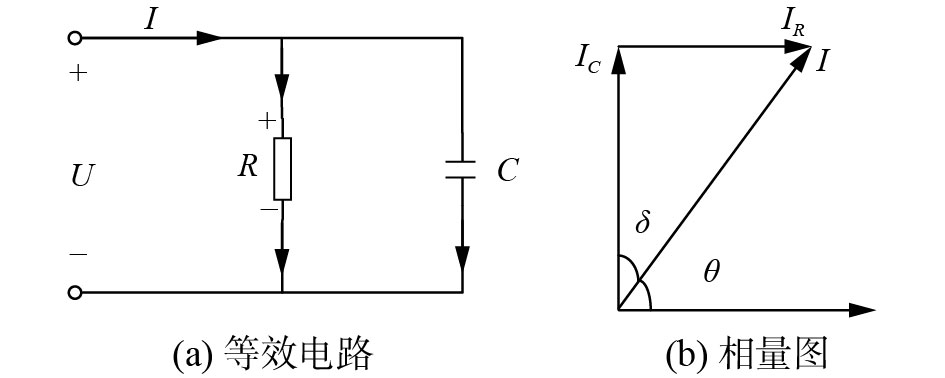Download: 图 1 电容设备的等效电路和相量图 Fig. 1 Capacitance equipment equivalent circuit and phasor diagram

 $\begin{split} {D_\delta }\left( t \right) = & {{\tilde U}_s}\left( t \right) - \tilde I\left( t \right) = - 2{\rm{sin}}\left( {\frac{\delta }{2}} \right){\rm{cos}}\left( {\omega t - \frac{{{\text{π} }}}{2}} \right) \end{split}$ (6)

 $R\left( \delta \right) = \frac{{{A_\delta }}}{\delta } = \frac{{2{\rm{sin}}\left( {\delta /2} \right)}}{\delta } = \frac{{{\rm{sin}}\left( {\delta /2} \right)}}{{\delta /2}}$ (7)

2 BP-SVM的Dδ(t)信号辨识方法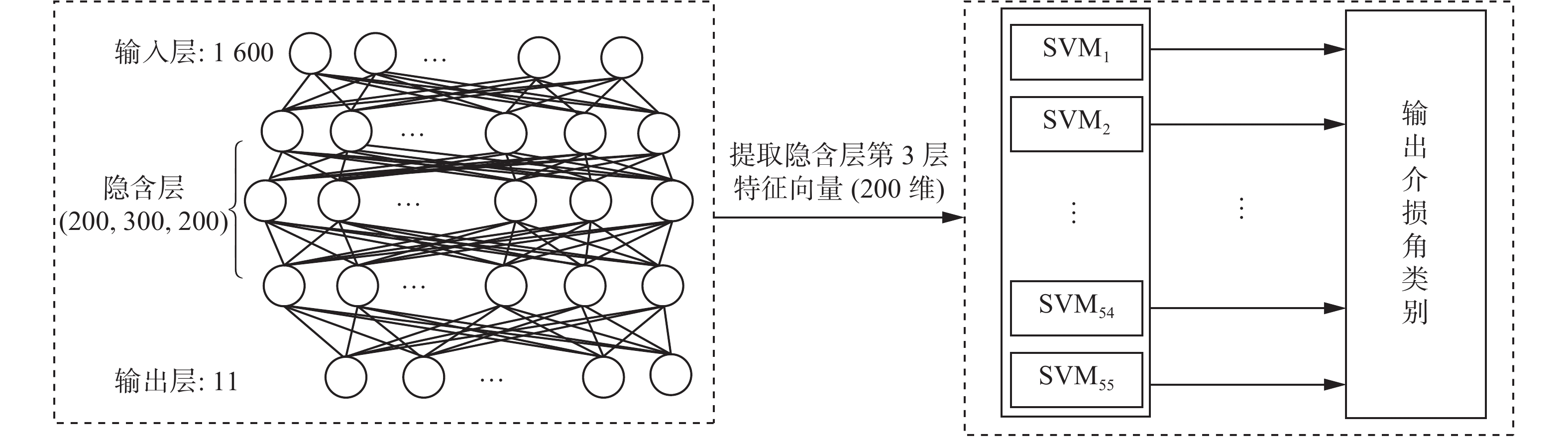Download: 图 2 BP-SVM模型 Fig. 2 BP-SVM model

1)假设目标电容器的介损角δ为3×10−4 rad，记作δ0，使用式(6)计算该电容器正常状态下的介损角表示信号 $D_{\delta_{0}}(t)$ t为采样间隔。

2)当δ0每次增加1×10−5 rad时，根据式(6)计算对应 $D_{\delta_{i}}(t)$ 的值，i∈{1,2, $\cdots$ ,10}，其中，介损角δ0Dδ(t)值记作 $D_{\delta_{0}}(t)$

3)分别对 $D_{{\delta_{0}}}(t)$ ~ $D_{\delta_{10}}(t)$ 加入一定强度的白噪声信号形成新的训练样本TrainSet，使用新的训练样本训练BP神经网络，提取特征后训练SVM模型。

4)使用训练好的BP神经网络模型和SVM模型辨识被测信号属于哪一类 $D_{\delta_{i}}(t)$ ，从而可以判断出当前被测信号的介损角为δi=δ0+10−5i

1)生成介损角δi为{3×10−4, 3.1×10−4,···, 3.9×10−4, 4×10−4} rad，频率分别为{49.50, 49.75, 50.00, 50.25,50.50}Hz条件下的介损角信号集 $D_{\delta_{i}} (t)$

2)对 $D_{\delta_{i}} (t)$ 按下列条件增加谐波：①谐波次数为3、5、7；②各次谐波的初始相角与基波相角差分别为 $- \displaystyle\frac{{\text{π}}}{3}{\text{、}}{\text{π}}{\text{、}}\displaystyle\frac{{\text{π}}}{3}$ ; ③各次谐波的幅值为基波幅值的0%、5%、10%。

3)所有的训练样本加入{20, 25, 30, 35}dB的4个等级的白噪声，每个等级的白噪声等级生成10个加噪信号形成新的训练集TrainSet。

4)在构建训练集方法的基础上，按照以下3个条件构建测试集TestSet：

①对各次谐波相角增加 $- \displaystyle\frac{\text{π}}{6} \sim \frac{\text{π}}{6}$ 的随机漂移；

③频率增加{−0.5, −0.25, 0, 0.25, 0.5}Hz的随机漂移；

④所有的测试样本加入{20, 25, 30, 35}dB的4个等级的白噪声，每个噪声等级生成5个加噪信号。

5)用构建好的训练样本集TrainSet训练本文的BP神经网络模型，图3为BP神经网络训练过程中的损失趋势。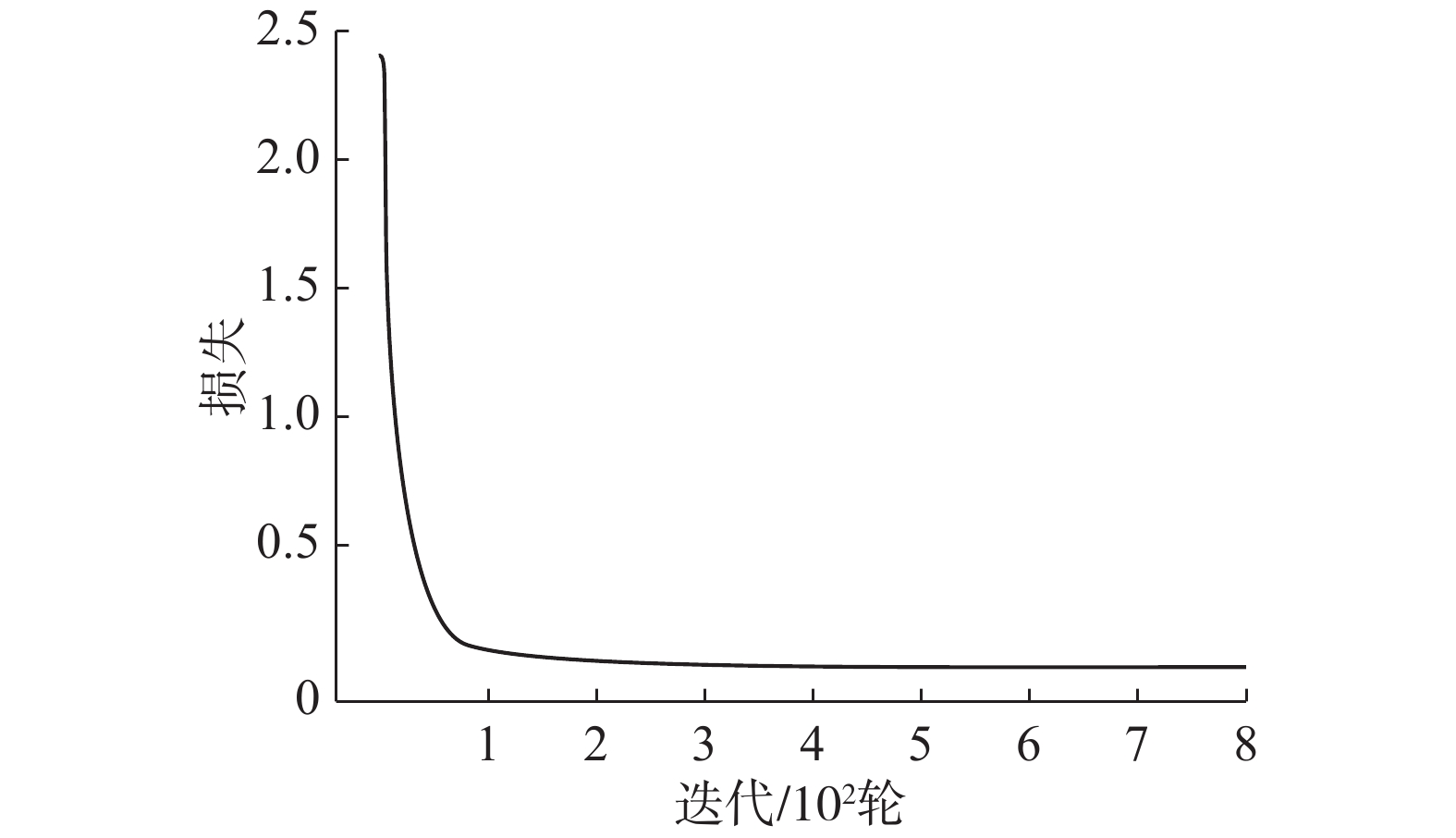Download: 图 3 BP神经网络的损失趋势 Fig. 3 BP neural network loss trend

6)基于5)中训练好的BP神经网络，将训练样本集TrainSet重新输入BP神经网络中，提取BP神经网络隐含层第3层的特征向量，将该特征向量和对应的类别标签组成新的训练样本SVMTrainSet，使用SVMTrainSet训练SVM模型。

7)使用TestSet检验本文提出的BP-SVM方法在不同δi下信号Dδi(t)的辨识能力，检验过程分为：①将TestSet输入训练好的BP神经网络中，提取隐含层第3层的特征向量；②将①中提取的特征向量作为SVM的输入，使用训练好的SVM对提取的特征向量进行分类，对介损角进行辨识。

3 实验结果与分析

3.1 介损角辨识结果表 1 BP-SVM和BP神经网络在测试集上的实验结果 Tab.1 BP-SVM and BP neural network experiment results on test sets表 2 BP-SVM和深度学习方法在测试集上的实验结果 Tab.2 BP-SVM and deep learning method experiment results on test sets表 3 BP-SVM在TestSet1上的实验结果 Tab.3 BP-SVM experiment results on Test Set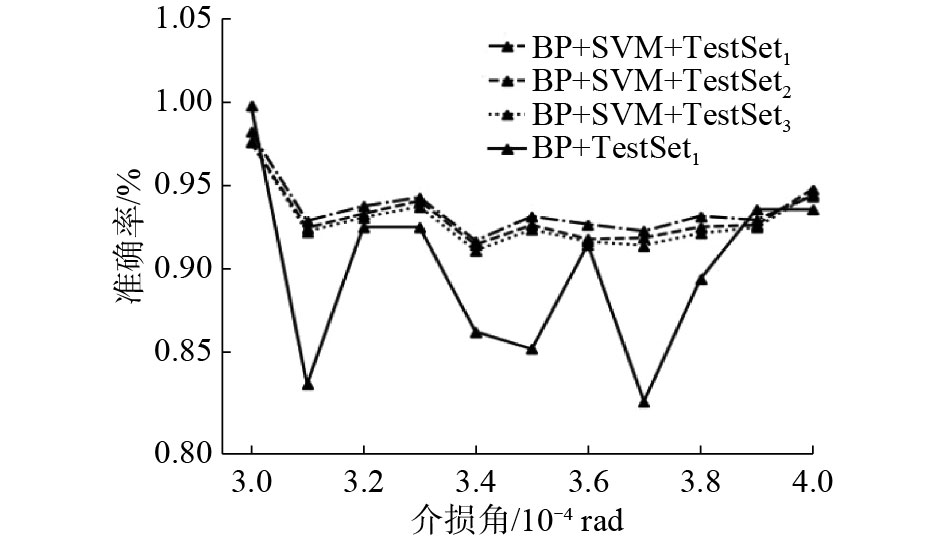Download: 图 4 不同模型和不同测试样本集的测试结果 Fig. 4 Test results for different models and test sets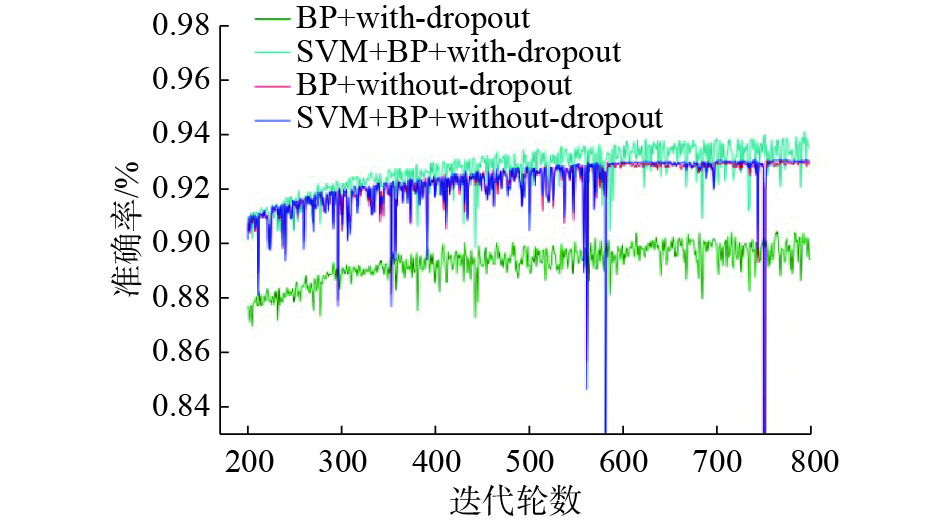Download: 图 5 有无dropout的测试结果 Fig. 5 Test results with or without dropout
3.2 白噪声和频率漂移对辨识结果的影响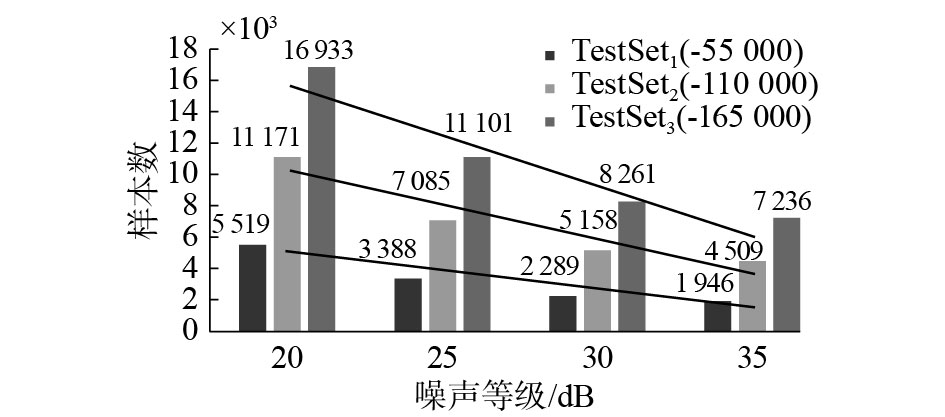Download: 图 6 不同等级噪声下的测试结果 Fig. 6 Test results at different levels of noise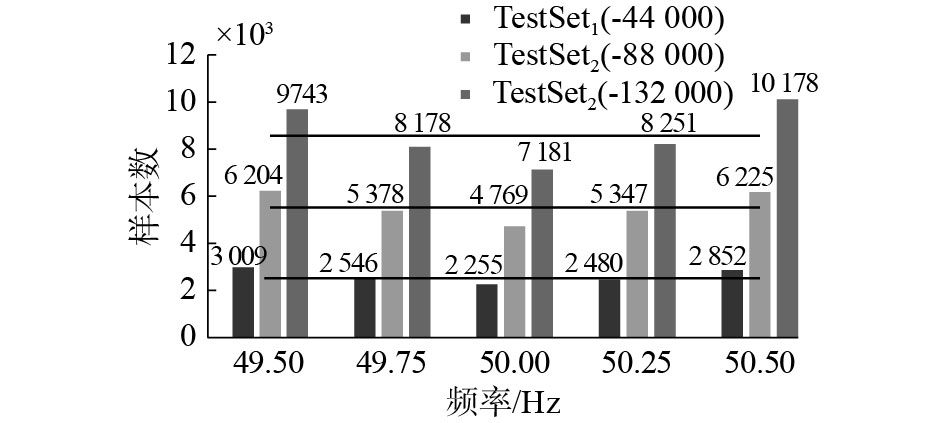Download: 图 7 不同频率下的测试结果 Fig. 7 Test results at different frequencies
4 结束语

1)本文所提出的BP-SVM方法具有更高的辨识准确率，并且在不同的测试样本集下均保持了较高的辨识准确率，模型具有更好的泛化能力。

2)本文所提出的BP-SVM方法比基于深度学习的方法具有更强的抗频率变化能力，频率的变化对于本文算法的性能无明显影响。

3)本文所提出的模型在较少的训练样本集下取得了较高的辨识准确率，当测试样本集3倍于训练样本集时，本文方法依旧将电容器介损角的辨识准确率保持在93.40%。

  李天云, 袁明哲, 蔡国伟, 等. 基于随机子空间和最小二乘法的介质损耗角检测方法[J]. 高电压技术, 2011, 37(4): 828-833. LI Tianyun, YUAN Mingzhe, CAI Guowei, et al. Measurement algorithm of dielectric loss angle based on SSI-LS[J]. High voltage engineering, 2011, 37(4): 828-833. (0)  王晓辉, 朱永利, 王艳, 等. 基于深度学习的电容器介损角在线辨识[J]. 电工技术学报, 2017, 32(15): 145-152. WANG Xiaohui, ZHU Yongli, WANG Yan, et al. Online Identification method of power capacitor dielectric loss angle based on deep learning[J]. Transactions of China electrotechnical society, 2017, 32(15): 145-152. (0)  董爽, 李天云, 王永, 等. 在线检测介质损耗角的矩阵束方法[J]. 电工技术学报, 2015, 30(18): 229-236. DONG Shuang, LI Tianyun, WANG Yong, et al. An On-line detection method of dielectric loss angle based on matrix pencil algorithm[J]. Transactions of China electrotechnical society, 2015, 30(18): 229-236. DOI:10.3969/j.issn.1000-6753.2015.18.028 (0)  WANG P, RGHUVEER M R, MCDERMID W, et al. A digital technique for the on-line measurement of dissipation factor and capacitance[J]. IEEE transactions on dielectrics and electrical insulation, 2001, 8(2): 228-232. DOI:10.1109/94.919938 (0)  尚勇, 杨敏中, 王晓蓉, 等. 谐波分析法介质损耗因数测量的误差分析[J]. 电工技术学报, 2002, 17(3): 67-71, 34. SHANG Yong, YANG Minzhong, WANG Xiaorong, et al. Error analysis for the dielectric loss factor measurement based on harmonic analysis[J]. Transactions of China electrotechnical society, 2002, 17(3): 67-71, 34. DOI:10.3321/j.issn:1000-6753.2002.03.015 (0)  刘伟, 黄新波, 章云. 电容型高压设备介损在线监测系统的现场采集单元设计[J]. 计算机测量与控制, 2010, 18(1): 233-236, 242. LIU Wei, HUANG Xinbo, ZHANG Yun. Design of field sampling unit of an on-line monitoring system of dielectric loss in capacitive high-voltage apparatus[J]. Computer measurement and control, 2010, 18(1): 233-236, 242. (0)  陈晓宇, 郑建勇, 梅军. 基于LC振荡频率变化量的电容器组早期故障在线智能预警方法及其实现[J]. 电力系统保护与控制, 2015, 43(5): 144-149. CHEN Xiaoyu, ZHENG Jianyong, MEI Jun. Power capacitor banks failure warning method based online intelligence LC oscillation frequency variation and its implementation[J]. Power system protection and control, 2015, 43(5): 144-149. (0)  袁飞, 杨震男, 卢毅. 一种基于相对比较法测量容性设备介质损耗的滤波算法[J]. 电力系统保护与控制, 2015, 43(19): 95-100. YUAN Fei, YANG Zhennan, LU Yi. A filtering algorithm for measuring capacitive device dielectric loss based on relative comparison method[J]. Power system protection and control, 2015, 43(19): 95-100. DOI:10.7667/j.issn.1674-3415.2015.19.016 (0)  王楠, 律方成, 梁英, 等. 基于高精度DFT的介损数字测量方法[J]. 高电压技术, 2003, 29(4): 3-5, 8. WANG Nan, LV Fangcheng, LIANG Ying, et al. Digital measurement of dielectric loss based on high accuracy DFT algorithm[J]. High voltage engineering, 2003, 29(4): 3-5, 8. DOI:10.3969/j.issn.1003-6520.2003.04.002 (0)  王微乐, 李福祺, 谈克雄. 测量介质损耗角的高阶正弦拟合算法[J]. 清华大学学报 (自然科学版), 2001, 41(9): 5-8. WANG Weile, LI Fuqi, TAN Kexiong. Higher-order sine fitting algorithm for dielectric loss measurement[J]. Journal of Tsinghua University (science and technology), 2001, 41(9): 5-8. (0)  冯小华, 张承学. 修正的谐波分析法可提高介损在线测量准确度[J]. 高电压技术, 2004, 30(8): 36-37. FENG Xiaohua, ZHANG Chengxue. Modified harmonics analysis approach for increasing precision in on-line measuring tan δ [J]. High voltage engineering, 2004, 30(8): 36-37. DOI:10.3969/j.issn.1003-6520.2004.08.014 (0)  LI Q, ZHAO T, SIEW W H. Definition and digital algorithms of dielectric loss factor for condition monitoring of high-voltage power equipment with harmonics emphasis[J]. IEE proceedings-generation, transmission and distribution, 2005, 152(3): 309-312. DOI:10.1049/ip-gtd:20045043 (0)  张忠蕾, 李庆民, 陈鹏. 谐波条件下高压电气设备介质损耗因数的定义和数字化算法[J]. 高压电器, 2004, 40(5): 327-329, 332. ZHANG Zhonglei, LI Qingmin, CHEN Peng. Definition and digital algorithms for dielectric loss factor of high voltage power apparatus under harmonics condition[J]. High voltage apparatus, 2004, 40(5): 327-329, 332. DOI:10.3969/j.issn.1001-1609.2004.05.003 (0)  张介秋, 梁昌洪, 韩峰岩, 等. 介质损耗因数的卷积窗加权算法[J]. 电工技术学报, 2005, 20(3): 100-104. ZHANG Jieqiu, LIANG Changhong, HAN Fengyan, et al. Convolution window weighted algorithm for dielectric loss Factor[J]. Transactions of China electrotechnical society, 2005, 20(3): 100-104. DOI:10.3321/j.issn:1000-6753.2005.03.019 (0)  徐志钮, 律方成, 赵丽娟. 基于加汉宁窗插值的谐波分析法用于介损角测量的分析[J]. 电力系统自动化, 2006, 30(2): 81-85. XU Zhiniu, LÜ Fangcheng, ZHAO Lijuan, et al. Analysis of dielectric loss angle measurement by Hanning windowing interpolation algorithm based on FFT[J]. Automation of electric power systems, 2006, 30(2): 81-85. DOI:10.3321/j.issn:1000-1026.2006.02.015 (0)  段大鹏, 江秀臣, 孙才新, 等. 基于正交分解的介质损耗因数数字测量算法[J]. 中国电机工程学报, 2008, 28(7): 127-133. DUAN Dapeng, JIANG Xiuchen, SUN Caixin, et al. A novel algorithm of dielectric loss measurement based on orthogonal decomposition[J]. Proceedings of the CSEE, 2008, 28(7): 127-133. DOI:10.3321/j.issn:0258-8013.2008.07.021 (0)  陈楷, 胡志坚, 王卉, 等. 介损角的非同步采样算法及其应用[J]. 电网技术, 2004, 28(18): 58-61. CHEN Kai, HU Zhijian, WANG Hui, et al. Algorithm and application of dielectric loss angle based on asynchronous sampling[J]. Power system technology, 2004, 28(18): 58-61. DOI:10.3321/j.issn:1000-3673.2004.18.013 (0)  WANG Kang, TENG Zhaosheng, WEN He, et al. Fast measurement of dielectric loss angle with time-domain quasi-synchronous algorithm[J]. IEEE transactions on instrumentation and measurement, 2015, 64(4): 935-942. DOI:10.1109/TIM.2014.2362839 (0)  王永, 滕召胜, 李建闽, 等. 基于采样序列重构的高精度介损角测量方法[J/OL]. 电工技术学报, [2018-04-24]. https://doi.org/ 10.19595/j.cnki.1000-6753.tces.171699. WANG Yong, TENG Zhaosheng, LI Jianmin, et al. High accuracy dielectric loss angle measurement method based on sampling sequence reconstruction[J/OL]. Transactions of China electrotechnical society, [2018-04-24]. https://doi.org/10.19595/j.cnki.1000-6753.tces.171699. (0)